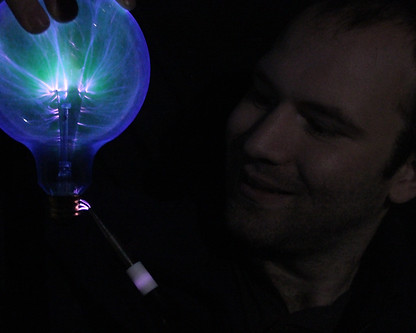top of page## High School Physics

• The student will analyze and explain how Newton’s Laws describe changes in an object’s motion.

• The student will analyze the behavior of forces.

• Friction, Gravity, Pulleys and Work​• The student will describe wave characteristics using both diagrams and calculations.

• Wave Generator

• The student will compare qualitatively how waves are propagated and transmit energy.

• Doppler Effect• The student will describe the types of electric charges and the forces that exist between them.

• The student will describe the sources and effects of electric and magnetic fields.bottom of page